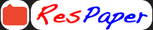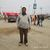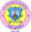Trending ▼   ResFinder# ISC Class XII Prelims 2019 : Physics (St. Peters School, RaeBareli)

5 pages, 55 questions, 24 questions with responses, 33 total responses,20Pawan Agnihotri St. Peter's School, RaeBareli
+Fave Message
 Home > stpeters1983 >   F Also featured on: School Page iscFormatting page ...

Half yearly Examination : September:2018 PHYSICS (Class 12 ) PAPER 1 (THEORY) (Maximum Marks: 70) (Time allowed: Three hours) (Candidates are allowed additional 15 minutes for only reading the paper. They must NOT start writing during this time.) All questions are compulsory. This question paper is divided in 4 Sections A, B, C and D as follows. Section A Question number 1 is of twelve marks. All parts of this question are compulsory. Section B Question numbers 2 to 12 carry 2 marks each with two questions having internal choice. Section C Question numbers 13 to 19 carry 3 marks each with two questions having internal choice. Section D Question numbers 20 to 22 are long-answer type questions and carry 5 marks each. Each question has an internal choice. Section A Question : 1(a) Objective type questions: 1. A constant current of 2.82 A flows in a resistor. The rms current is : (a) 2.82 A (b) 2.0 A (c) 1.41 A (d) undefined 2. Two thin lenses of optical powers of -10 D and + 6 D are placed in contact to each other. The focal length of the combination is : (a) + 0.25 cm (b) - 0.25 cm (c) + 0.25 m (d) -0.25 m 3. Point out the wrong statement : Electromagnetic waves (a) Are transverse (b) are produced by accelerating charges ( c ) travel with same speed in all media (d) travel with speed of light in free space. 4. A moving electric charge produces : (a) Both electric and magnetic fields. (b) Electric field only (c) Magnetic field only (d) Neither of these two fields. 5. The reactance of a capacitor in an AC circuit is 10 ohm. If the frequency is doubled the reactance will become: (a) 5 (b) 10 (c) 15 (d) 20 Question 1 (b): Answer all questions briefly and to the point: 1. An ideal inductor in an AC circuit consumes no power, though both V and I are non-zero. Why? 2. Arrange in ascending order of wavelength: microwaves, rays, radio waves, ultraviolet light. 3. Give the expression for the power factor in an C R circuit in terms of the resistance R and capacitive reactance . 4. The magnetic flux threading a coil changes from 12 10 Wb to 6.0 10 Wb in 0.01 sec. calculate the induced emf. 5. State Ampere s circuital law. 6. A 900pF capacitor is charged by a 100 V battery. How much energy is stored by the capacitor? 7. State three basic properties of charge. Section B Question 2: (a) Find the value of resistance X in the given circuit so that the junctions M and N are at the same potential.Formatting page ...

Top Contributors
to this ResPaperAshu(13)Isha Gandhi(6)Siddharth S(4)Allam(3)Formatting page ...Formatting page ...Formatting page ...Tags : ISC Physics Half yearly question paper 2018